## 机器学习——神经网络（四）：BP神经网络

2020/8/11 19:50:13 文章标签:

## BP神经网络（Back Propagation）

误差逆传播算法（error Back Propagation 简称BP），实际上是多层感知器的一种,在1986由Rumelhart和Hinton为首的科学小组提出。BP神经网络具有任意复杂的模式分类能力和优良的多维函数映射能力，解决了简单感知器不能解决的异或问题和其他问题。从结构而言具有输入层、隐含层和输出层；从本质上来讲是以网络误差平方为目标函数、采用梯度下降法来计算目标函数的最小值。BP神经网络结构

### 1. 主要过程：

• (1)工作信号向前正向传播的子过程
• (2)误差信号反向传播向后反馈的子过程
借用一个例子BP（Back Propagation）神经网络学习笔记：我要追求女神，那我总得表示一下吧！于是我给她买花，讨她欢心。然后，她给我一些表示（或者叫暗示），根据这个表示，与我最终目的进行对比（追求到女神），然后我进行调整继续表示，一直循环往复，知道实现最终目的——成功最求到女神。我的表示就是“信号前向传播”，女神的表示就是“误差反向传播”。这就是BP神经网络的核心。
• 其中主要的过程是：
正向传播获得输出结果
BP正向传播

BP反馈反向传播

• 学习目的：

• 学习方式：

• 学习本质：

• 学习核心：

### 3. 算法详解

神经网络模拟生物的神经结构与活动，构造分类器。基本组成单位为神经元，当输入神经元的参量大于某一个阈值的时候，神经元变为兴奋状态，产生输出，否则不响应。这个输入与其相连接的所有神经元都有关系，神经元的响应函数可以分为多种不同形式的（即激活函数）。

1. 激活函数
2. BP推导

1）激活函数

（1）sigmoid函数

$f(z) = \frac{1}{1+e^{-z}}$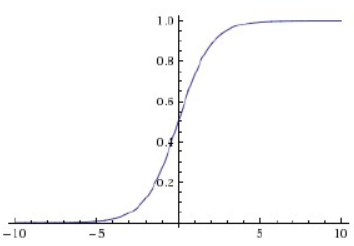sigmoid 函数及其导数图像

• 在深度神经网络中梯度反向传播时导致梯度爆炸和梯度消失
• sigmoid的输出output不是0均值（zero-centerd）
• 解析式中含有幂运算，计算求解的代价较高，对于大规模的机器学习算法和训练过程对时间空间的消耗较高

（2）tanh函数

$tanh(x) = \frac{e^x - e^{-x}}{e^x+e^{-x}}$
tanh函数及其导数图像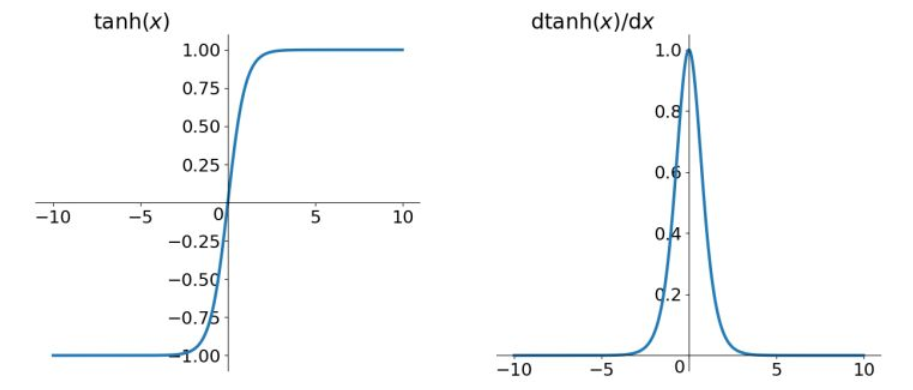tanh 函数及其导数图像

• 解决了Sigmoid函数不是zero-centered输出的问题

• 梯度消失和幂运算的问题仍然存在

（3）Relu函数
Relu函数解析式
$Relu = max(0,x)$
Relu函数及其导数图像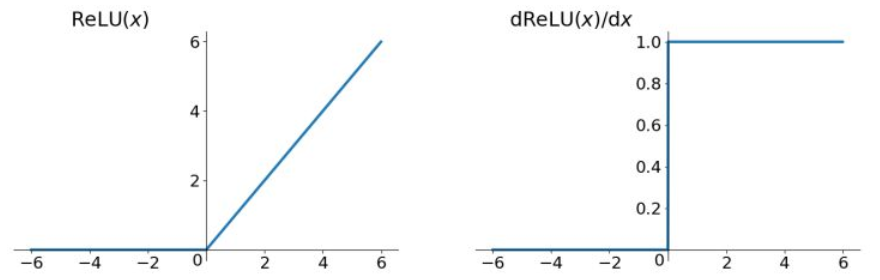Relu 函数及其导数图像

• 解决了梯度消失（gradient vanishing）的问题（在正区间）
• 不含有幂指数计算，计算速度快
• 收敛速度较快

• Relu的输出不是zero-centered的值
• Deep ReLU Problem,即有的神经元可能永远不会被激活，导致相应的参数永远不会被更新（主要原因：
（1）：初始化参数较差，发生的几率很小；
（2）learning rate太高）

（4）Leaky ReLU函数（目前使用最为广泛和通用的activation function

$f(x) = max(ax,x)$
Leaky ReLU函数及其导数的图像：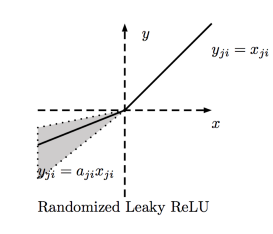Leakey Relu 函数图像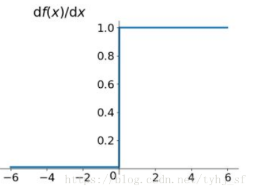Leakey Relu 函数导数图像

（5）ELU（exponential Linear Units）函数

${f(x)}=\left\{ \begin{array}{rcl} x && {if x > 0}\\ a（e^x-1)） && { otherwise} \end{array} \right.$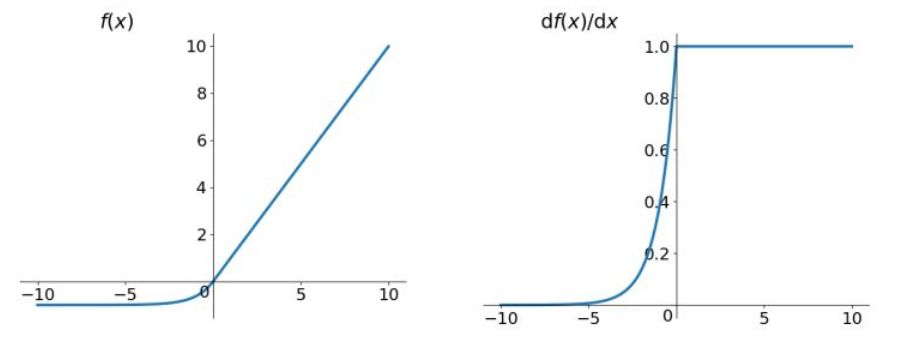ELU函数及其导数

2） BP推导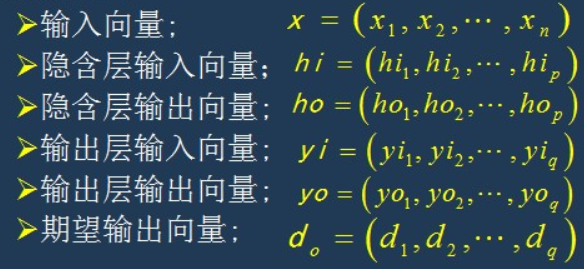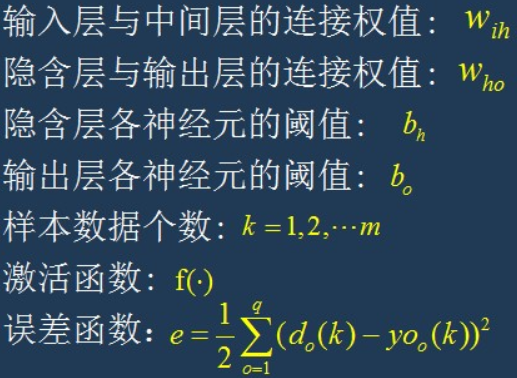1.网络初始化：

1. 随机选择第k个输入样本和其对应的期望输出（即标签结果）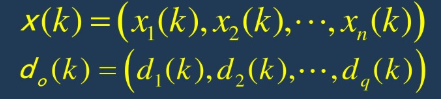1. 计算隐含层的各个神经元的输入和输出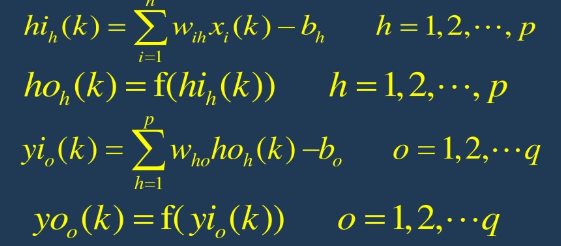1. 利用网络期望输出和实际输出，计算误差函数e对输出层的各神经元的偏导数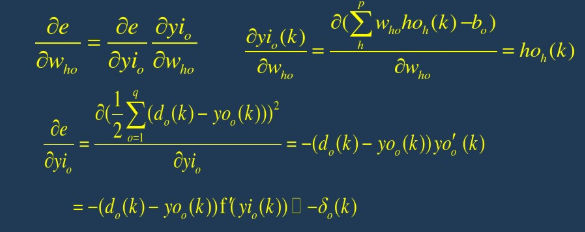1. 利用隐藏层到输出层的连接权值、隐含层的输出值、输出层的偏导数计算出误差函数对隐含层各神经元的偏导数
1. 根据输出层各神经元的$\delta_o(k)$和隐含层各神经元的输出来修正连接权值$w_{ho}(k)$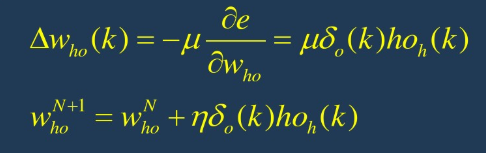1. 利用隐含层各神经元的$\delta_o(k)$和输入层的输出层的连接权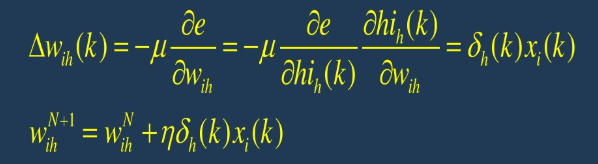1. 计算全局误差：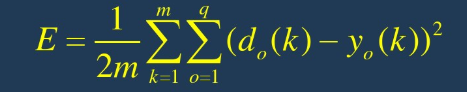1. 判断误差或者迭代次数是否满足要求，当误差在一定的精度范围之内或者达到迭代次数结束循环。否则返回到第三步重复进行下一轮学习。

### 4. 代码实现

import math
import random
import numpy as np
import matplotlib.pyplot as plt
random.seed(0)  # random加种子，让每次生成的随机数都是相同的
def rand(a, b):
'''随机函数'''
return (b - a) * random.random() + a

def make_matrix(m, n, fill=0.0):
# 方法1,：直接使用numpy的zeros函数
return np.zeros([m,n]).tolist()

#方法2：定义list，逐行加入
#     mat = []
#     for i in range(m):
#         mat.append([fill] * n)
#     return mat

def sigmoid(x):
'''sigmoid激活函数'''
return 1.0 / (1.0 + math.exp(-x))

def sigmoid_derivative(x):
'''sigmoid函数的导数'''
return x * (1 - x)

class BPNeuralNetwork:
def __init__(self):
self.input_n = 0           # 初始化输入层神经元数
self.hidden_n = 0          # 初始化隐含层神经元数
self.output_n = 0          # 初始化输出层神经元数
self.input_cells = []      # 初始化输入层神经元
self.hidden_cells = []     # 初始化隐含层神经元
self.output_cells = []     # 初始化输出层神经元
self.input_weights = []    # 输入层到隐含层的权重
self.output_weights = []   # 隐含层到输出层的权重
self.input_correction = [] # 输入层校正值
self.output_correction = [] # 输出层的校正值

def setup(self, ni, nh, no):
'''
ni——输入层神经元的个数
nh——隐含层神经元的个数
no——输出层神经元的个数

'''
self.input_n = ni + 1      #加上一列偏置值
self.hidden_n = nh
self.output_n = no
# init cells
self.input_cells = [1.0] * self.input_n   # 初始化1行ni+1列的单位矩阵
self.hidden_cells = [1.0] * self.hidden_n # 初始化1行nh列的单位矩阵
self.output_cells = [1.0] * self.output_n # 初始化1行no列的单位矩阵
# 初始化输入层到隐含层之间的权重
self.input_weights =(np.random.random([self.input_n,self.hidden_n])-0.8)
# 初始化输入层到隐含层之间的权重
self.output_weights =(np.random.random([self.hidden_n,self.output_n]))*2
# 初始化校正矩阵
self.input_correction = make_matrix(self.input_n, self.hidden_n)
self.output_correction = make_matrix(self.hidden_n, self.output_n)

def predict(self, inputs):
# 激活输出层神经元
for i in range(self.input_n - 1):
self.input_cells[i] = inputs[i]
# 激活隐含层神经元
for j in range(self.hidden_n):
total = 0.0
for i in range(self.input_n):
total += self.input_cells[i] * self.input_weights[i][j]
self.hidden_cells[j] = sigmoid(total)
# 激活输出层神经元（即输出结果）
for k in range(self.output_n):
total = 0.0
for j in range(self.hidden_n):
total += self.hidden_cells[j] * self.output_weights[j][k]
self.output_cells[k] = sigmoid(total)
return self.output_cells[:]

def back_propagate(self, case, label, learn, correct):
'''
case——输入数据
label——标签数据
learn——学习率
correct——校正参数
'''
# 正向传参
self.predict(case)
# 获取输出层误差及误差相对于输出层神经元的偏导数
output_deltas = [0.0] * self.output_n
for o in range(self.output_n):
error = label[o] - self.output_cells[o]
output_deltas[o] = sigmoid_derivative(self.output_cells[o]) * error
# 获取隐含层的相对误差及其相对于隐含层神经元的偏导数
hidden_deltas = [0.0] * self.hidden_n
for h in range(self.hidden_n):
error = 0.0
for o in range(self.output_n):
error += output_deltas[o] * self.output_weights[h][o]
hidden_deltas[h] = sigmoid_derivative(self.hidden_cells[h]) * error
# 更新隐含层到输出层的权值
for h in range(self.hidden_n):
for o in range(self.output_n):
change = output_deltas[o] * self.hidden_cells[h]
self.output_weights[h][o] += learn * change + correct * self.output_correction[h][o]
self.output_correction[h][o] = change
# 更新输入层到输出层的权重
for i in range(self.input_n):
for h in range(self.hidden_n):
change = hidden_deltas[h] * self.input_cells[i]
self.input_weights[i][h] += learn * change + correct * self.input_correction[i][h]
self.input_correction[i][h] = change
# 获取全局误差
error = 0.0
for o in range(len(label)):
error += 0.5 * (label[o] - self.output_cells[o]) ** 2
return error

def train(self, cases, labels, limit=10000, learn=0.05, correct=0.1):
for j in range(limit):
error = 0.0
for i in range(len(cases)):
label = labels[i]
case = cases[i]
error += self.back_propagate(case, label, learn, correct)
if j%100==0:
plt.scatter(j,error)
plt.title('Error curve')
plt.xlabel('iteration')
plt.ylabel('error')
plt.show()

def test(self):
cases = [
[0, 0],
[0, 1],
[1, 0],
[1, 1],
]
labels = [, , , ]
self.setup(2, 5, 1)
self.train(cases, labels, 10000, 0.05, 0.1)
for case in cases:
print(self.predict(case))

if __name__ == '__main__':
nn = BPNeuralNetwork()
nn.test()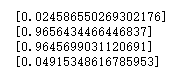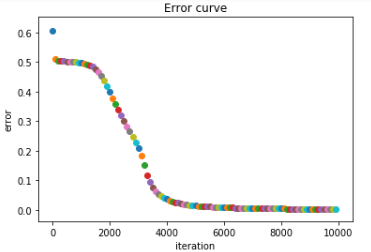>

暂无相关的数据...